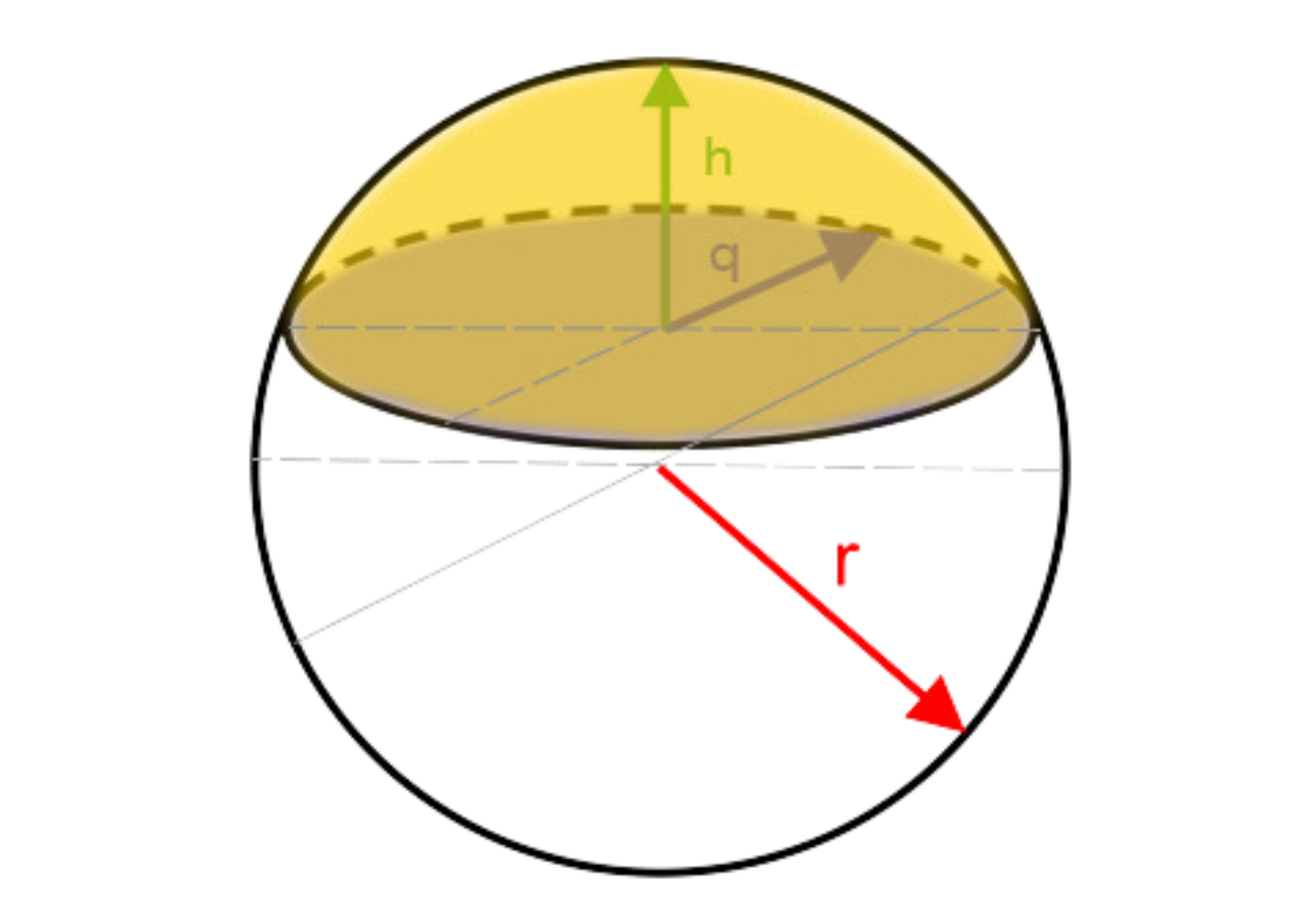﻿ Spherical cap: - on line calculation, formula - FORMIAX

# Spherical cap - surface and volumeS =  π. (q2 + 2.r.h)

V = 1/3.π.h2.(3r – h)

S = surface area

V = volume

h = height

WHAT IS IT ?
A spherical cap is the region of a sphere which lies above (or below) a given plane. If the plane passes through the center of the sphere, the cap is a called a Spherical cap

CALCULATION:

Enter unit e.g.: inch

Round to number of decimals places

YOU MIGHT BE INTERESTED: